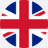# Andhra university cgpa to percentage calculator

Andhra University is a prestigious institution located in the state of Andhra Pradesh, India. It follows a CGPA (Cumulative Grade Point Average) grading system to evaluate the performance of its students. However, the grading system can be confusing, and students often find it challenging to calculate the percentage from their CGPA. In this article, we will discuss the process of converting CGPA to percentage for Andhra University students.

Also check: How to Convert CGPA to Percentage?

## Andhra University CGPA to Percentage Conversion Formula

The formula for converting CGPA to percentage is relatively easy and accurate. All you need to do is multiply your CGPA by 10. For example, if your CGPA is 8.5, your percentage score will be 8.5 x 10 = 85%.

Percentage Score = CGPA x 10

Also read: Calculate percentage of marks

Andhra University follows a 10-point grading system, where the maximum grade point is 10, and the minimum grade point is 0. The grading system is as follows:

## Andhra University PG CGPA to Percentage Calculator:

Students can use the CGPA to Percentage Calculator below to convert their CGPA to percentage quickly and accurately. The calculator requires the student to input their CGPA, and the calculator will provide the percentage of marks in real time. Students can use this calculator to convert their CGPA for various purposes like admission, scholarship, or job application.

## Andhra University CGPA to Percentage Conversion Certificate:

Students who require an Andhra University CGPA to Percentage Conversion Certificate can obtain the same from the university. The application for the conversion certificate can be downloaded from the official website of Andhra University. The application form must be filled in with the required details, and a copy of the mark sheet or transcript should be attached. The application form and the prescribed fee must be submitted to the Controller of Examinations to obtain the conversion certificate.

Andhra University follows a 10-point grading system to evaluate students' academic performance. To convert the CGPA to percentage, students can use the formula CGPA x 9.5. The Andhra University PG CGPA to Percentage Calculator can be used to convert the CGPA to percentage quickly and accurately. Students who require an Andhra University CGPA to Percentage Conversion Certificate can obtain the same from the university by submitting the application form with the prescribed fee.

Related pages,

## FAQ’s:

### Q: How can I calculate my CGPA in Andhra University?

A: To calculate your CGPA in Andhra University, you need to first determine your grade points for each subject. Then, multiply the grade point for each subject by the number of credits for that subject. Add up the total grade points for all subjects and divide by the total number of credits. The resulting number is your CGPA.

### Q: How does the Andhra University grading system work?

A: In the Andhra University grading system, marks are assigned a letter grade from O to F based on the range of marks. O represents outstanding performance, A represents excellent performance, B represents very good performance, C represents good performance, D represents satisfactory performance, E represents pass performance, and F represents fail performance.

### Q: What is the minimum CGPA required to pass in Andhra University?

A: In Andhra University, the minimum CGPA required to pass is 5.0.

### Q: Can I convert my Andhra University CGPA to percentage using a different formula?

A: No, the formula for converting Andhra University CGPA to percentage is fixed at CGPA * 10. Using a different formula may result in incorrect percentage values.

### Q: How can I obtain an Andhra University CGPA to percentage conversion certificate?

A: You can obtain an Andhra University CGPA to percentage conversion certificate by contacting the Office of the Controller of Examinations at the university. They will provide you with a certificate that includes your CGPA and percentage based on the universitys conversion formula.£
GBP